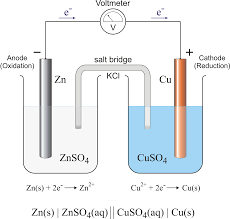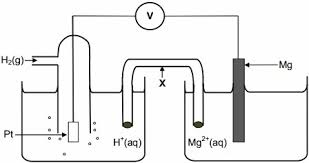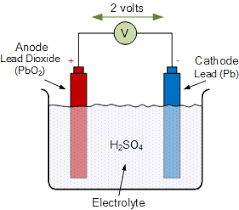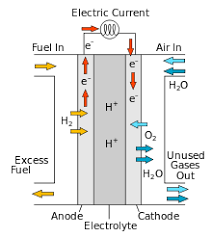# Edexcel Chemistry - Topic 14: Redox II

• Created by: Ryan C-S
• Created on: 11-04-18 21:26

## Oxidising and Reducing Agents

• Oxidising Agents are Electron Acceptors e.g. Cl2 and end up being reduced
• Reducing Agents are Electron Donors e.g. Mg and end up being oxidised
• Fluorine is the best oxidising agent as it has the greatest tendancy to gain electrons due to its small size and high nuclear attraction
• Caesium is the best reducing agent as it has the greatest tendancy to lose electrons due to its large size and high shielding which create a weaker nuclear attraction
• Francium would be a stronger reducing agent but it unstable
1 of 14

## Electrochemical Cells

• An electrochemical cell consists of two half cells connected by a salt bridge to generate a potential difference (voltage)
• Half cells consist of a metal electrode and a solution of a compound containing the metal e.g. Cu and CuSO4
• A potential difference forms when an external circuit is complete because one metal has a greater tendendcy to oxidise and release electrons than the other causing a build up of electrons on one electrode
e.g. Zn has a greater tendency than Cu to oxidise so releases electrons establishing a p.d.
• The salt bridge is used to connect up the circuit. The free moving ions conduct the charge
• The salt bridge should be unreactive with the electrodes and solution
• KNO3 is often used for this as opposed to KCl as the Chloride ions can form complexes with Cu2+ ions
• A high resistance voltmeter is used to measure the voltage. A high resistance voltmeter must be used in order to stop the current flowing in the curcuit as this prevents the reactants from being used up
Cu2+(aq) + 2e- --> Cu(s): Positive Electrode; electrons used up
Zn(s) --> Zn2+(aq) + 2e- : Negative Electrode; electrons given off
2 of 14

## Electrochemical Cell Diagram3 of 14

## Cell Diagrams

• Electrochemical cells can be represented by a cell diagram:
Zn(s) | Zn2+(aq) || Cu2+(aq) | Cu(s)
• If a system doesn't include a metal that can act as an electrode, then a platinum electrode is used and included in the cell diagram
• A platinum electrode is used because it is unreactive and can conduct electricity
e.g. for the reaction Fe2+(aq) --> Fe3+(aq) + e- there is no solid conducting surface so a platinum electrode is used - || Fe3+(aq), Fe2+(aq) | Pt
• A cell diagram the more oxidised species is closest to the || with the oxidised and reduced species seperated by a comma
• If a half equation has multiple physical states then the solid vertical lines should be used between each state boundary
e.g. Cl2(g) + 2e- --> 2Cl-(aq) ; || Cl2(g) | Cl-(aq) | Pt(s)
4 of 14

## Measuring Electrode Potentials

• The absolute potential of a half cell must be calculated by measuring the potential difference between two electrodes
• One of the half cells must have a known potential so the potential difference can between the two half cells can be measured
• Each electrode is assigned a relative potential by linking it to a reference electrode (Standard Hydrogen Electrode) which is given a potential of zero volts
H2(g) ⇌ 2H+(aq) + 2e-
Pt(s) | H2(g) | H+(aq) ||
• Platinum wire coated in porous platinum black (which acts as a catalyst) to allow the equilibrium to be achieve
Standard Conditions are:
• Temperature: 298K
• Pressure: 100kPa
• Solutions: 1.00mol dm-3
• No current flowing
5 of 14

## Standard Hydrogen Electrode

H2(g) ⇌ 2H+(aq) + 2e-

Pt(s) | H2(g) | H+(aq) || Mg2+(aq) | Mg(s)6 of 14

## Standard Electrode Potentials

• Standard conditions are required because the position of the redox equilibrium will change with conditions
• The Standard Hydrogen Electrode is difficult to use so a different easier electrode is used and calibrated against the Standard Hydrogen Electrode. These are called Secondary Standards
• A common secondary standards include Silver|Silver Chloride
• When an electrode system is connected to a standard hydrogen electrode, the potential difference measured is the standard electrode potential
• Standard Electrode Potentials are found in data books with the more oxidised form on the left

Li+(aq) | Li(s) E = -3.03V

Li+(aq) + e- ⇌ Li(s) E = -3.03V

7 of 14

## Calculating Electromotive Force

• Electromotive Force (emf) is a measure of the driving force of the whole cell i.e. the amount of work that is obtainable from the whole cell.
• Internal resistance means that if a current flows, the potential difference or voltage obtained will be less than the emf
• More Negative half equations will oxidise (<--)
More Positive half equations will reduce (-->)
• Ecell (emf) = Ered - Eox
• The electrochemical series arranges redox equilibria in order of their standard electrode potentials
• The more powerful reducing agents will be found at the most negative end of the electrochemical series and vice versa
• The more negative electrodes will oxidise and go from left to right and allows a reactions feasibility to be determined
• If the overall potential is positive, the reaction will be thermodynamically feasible
8 of 14

## Effect of Changing Conditions on Redox Equilibria

• The effects of changing conditions on Ecell can be made by applying Le Chateliers Principle
• Ecell is a measure of how far from the equilibrium the cell reaction lies. The more positive the Ecell, the more likely a reaction is to occur
• If current is allowed to flow, the cell reaction will occur and the Ecell will fall to zero as the reaction will proceed until the reactant concentrations drop
• If non standard conditions are used, a reaction may or may not go to completion

Concentration

• Increasing concentrations of reactants would increase the Standard Electrode Potential due to Le Chateliers Principle

Temperature

• Most cells are exothermic in the spontaneous direction so applying Le Chateliers principle to a temperature rise would result in a decrease in Standard Electrode Potential because the equilibrium would shift backwards in the endothermic direction
9 of 14

• Rechargeable battery cells used to power the starter motor or lights in a car
• PbSO4 + 2e- --> Pb + [SO4]2-  E = -0.036V
• PbO2 + [SO4]2- + 4H+ + 2e- --> PbSO4 + 2H2O  E = +1.69V
• PbO2 + Pb + 2[SO4]2- + 4H+ --> 2PbSO4 + 2H2O  E = +2.04V10 of 14

## Lithium Battery

• Non-rechargeable Battery cells that power laptops and mobile phone batteries
• +ve Electrode: Li+ + MnO2 + e- --> LiMnO2
• -ve Electrode: 2Li --> 2Li+ + 2e-
• Li(s) | Li+(aq) || Li+(aq) | MnO2(s), LiMnO2(s) | Pt(s)
• Lithium ions act as an electrolyte11 of 14

## Hydrogen Fuel Cell

• Hydrogen fuel cells are a renewable source of energy that maintains a constant voltage
• +ve Electrode: 0.5 O2(g) + 2H+ + 2e- --> H2O(l)
• -ve Electrode: H2(g) --> 2H+ + 2e-
• Overall Reaction: H2(g) + 0.5 O2(g) --> H2O(l)
• Hydrogen Fuel Cells are less polluting (releasing only water) and have a greater efficiency than standard electrochemical cells but are expensive, storing hydrogen is difficult and they have a limited lifetime so need regular replacement12 of 14

## Disproportionation Reactions

• A disproportionation reaction is one where an element (species) is simulatenously oxidised and reduced
• Copper(I) Iodide when reacting with sulphuric acid will disproportionate to Cu2+ and Cu metal
• Cu+(aq) + e-  --> Cu(s)  E = +0.52V
• Cu2+(aq) + e-  --> Cu+(aq) E = +0.15V
• E = +0.52 - +0.15 = + 0.37V
• If the overall potential has a positive value, the reaction will be thermodynamically feasible and disproportionation will occur
• A reaction may be thermodynamically feasible but may have too high an activation energy or have a slow rate of reaction and may not go
13 of 14

## Redox Titrations

[MnO4]-(aq) + 8H+(aq) + 5Fe2+(aq) --> Mn2+(aq) + 4H2O(l) + 5Fe3+(aq)

End Point: Colourless --> Pink/Purple

Method

• Add a known concentration of Fe2+ in FeSO4 to a conical flask using a pipette
• Pipette excess H+ ions in sulphuric acid into the flask
• Fill a burette with KMnO4
• Open the tap over the flask and start swirling the flask
• When the end point has been reached, turn off the tap
• Record the change in levels on the burette to find out how much of the KMnO4 was used
• Repeat until two concordant results have been achieved
• Calculate the number of moles used and then the molarity (concentration) of the solution
14 of 14